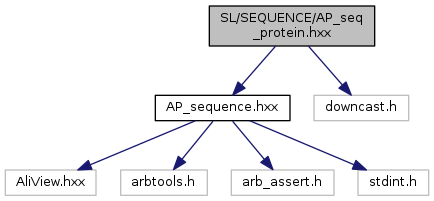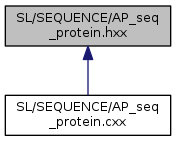ARB
AP_seq_protein.hxx File Reference
`#include <AP_sequence.hxx>`
`#include <downcast.h>`
Include dependency graph for AP_seq_protein.hxx:This graph shows which files directly or indirectly include this file:Go to the source code of this file.

## Classes

class  FINAL_TYPE< SEQTYPE >
xml element More...

## Enumerations

enum  AP_PROTEINS {
APP_ILLEGAL = 0, APP_A = (1 << 0), APP_C = (1 << 1), APP_D = (1 << 2),
APP_E = (1 << 3), APP_F = (1 << 4), APP_G = (1 << 5), APP_H = (1 << 6),
APP_I = (1 << 7), APP_K = (1 << 8), APP_L = (1 << 9), APP_M = (1 << 10),
APP_N = (1 << 11), APP_P = (1 << 12), APP_Q = (1 << 13), APP_R = (1 << 14),
APP_S = (1 << 15), APP_T = (1 << 16), APP_V = (1 << 17), APP_W = (1 << 18),
APP_Y = (1 << 19), APP_STAR = (1 << 20), APP_GAP = (1 << 21), APP_X = (APP_GAP-1),
APP_DOT = APP_X | APP_GAP, APP_B = APP_D | APP_N, APP_Z = APP_E | APP_Q, APP_J = APP_I | APP_L,
APP_MAX = (APP_GAP<<1)-1
}

## Enumeration Type Documentation

 enum AP_PROTEINS
Enumerator
APP_ILLEGAL
APP_A
APP_C
APP_D
APP_E
APP_F
APP_G
APP_H
APP_I
APP_K
APP_L
APP_M
APP_N
APP_P
APP_Q
APP_R
APP_S
APP_T
APP_V
APP_W
APP_Y
APP_STAR
APP_GAP
APP_X
APP_DOT
APP_B
APP_Z
APP_J
APP_MAX

Definition at line 21 of file AP_seq_protein.hxx.